About Contribute Source main/trg/

# TRG Algorithm

The TRG algorithm, which stands for tensor renormalization group is a strategy for evaluating a fully contracted network of tensors by decimating the network in a heirarchical fashion. The strategy is to factorize each tensor in the network using a truncated singular value decomposition (SVD) into two smaller factor tensors. Then each factor tensor is contracted with another factor from a neighboring tensor, resulting in a new contracted lattice of half as many tensors.

The term “renormalization group” is a term used in the physics literature to refer to processes where less important information at small distance scales is repeatedly discarded until only the most important information remains.

## Motivation — Ising Model

TRG can be used to compute certain large, non-trivial sums by exploiting the fact that they can be recast as the contraction of a lattice of small tensors.

A classic example of such a sum is the “partition function” $Z$ of the classical Ising model at temperature T, defined to be

@@ Z = \sum_{\sigma_1 \sigma_2 \sigma_3 \ldots} e^{-E(\sigma_1,\sigma_2,\sigma_3,\ldots)/T} @@

where each Ising “spin” $\sigma$ is just a variable taking the values $\sigma = +1, -1$ and the energy $E(\sigma_1,\sigma_2,\sigma_3,\ldots)$ is the sum of products $\sigma_i \sigma_j$ of neighboring $\sigma$ variables. In the two-dimensional case described below, there is a “critical” temperature $T_c=2.269\ldots$ at which this Ising system develops an interesting hidden scale invariance.

### Background: Ising Model in One dimension

In one dimension, spins only have two neighbors since they are arranged along a chain. For a finite-size system of N Ising spins, the usual convention is to use periodic boundary conditions meaning that the Nth spin connects back to the first around a circle: @@ E(\sigma_1,\sigma_2,\sigma_3,\ldots,\sigma_N) = \sigma_1 \sigma_2 + \sigma_2 \sigma_3 + \sigma_3 \sigma_4 + \ldots + \sigma_N \sigma_1 \:. @@

The classic “transfer matrix” trick for computing $Z$ goes as follows: @@ Z = \sum_{\{\sigma\}} \exp \left(\frac{-1}{T} \sum_n \sigma_n \sigma_{n+1}\right) = \sum_{\{\sigma\}} \prod_{n} e^{-(\sigma_n \sigma_{n+1})/ T} = \text{Tr} \left(M^N \right) @@

where $\text{Tr}$ means “trace” and the transfer matrix $M$ is a 2x2 matrix with elements

@@ M_{\sigma^{\\!} \sigma^\prime} = e^{-(\sigma^{\\!} \sigma^\prime)/T} \ . @@

Pictorially, we can view $\text{Tr}\left(M^N\right)$ as a chain of tensor contractions around a circle:With each 2-index tensor in the above diagram defined to equal the matrix M, it is an exact rewriting of the partition function $Z$ as a tensor network.

For this one-dimensional case, the trick to compute $Z$ is just to diagonalize $M$. If $M$ has eigenvalues $\lambda_1$ and $\lambda_2$, it follows that $Z = \lambda_1^N + \lambda_2^N$ by the basis invariance of the trace operation.

### Two dimensional Ising Model

Now let us consider the main problem of interest. For two dimensions, the energy function can be written as @@ E(\sigma_1, \sigma_2, \ldots) = \sum_{\langle i j \rangle} \sigma_i \sigma_j @@ where the notation $\langle i j \rangle$ means the sum only includes $i,j$ which are neighboring sites. It helps to visualize the system: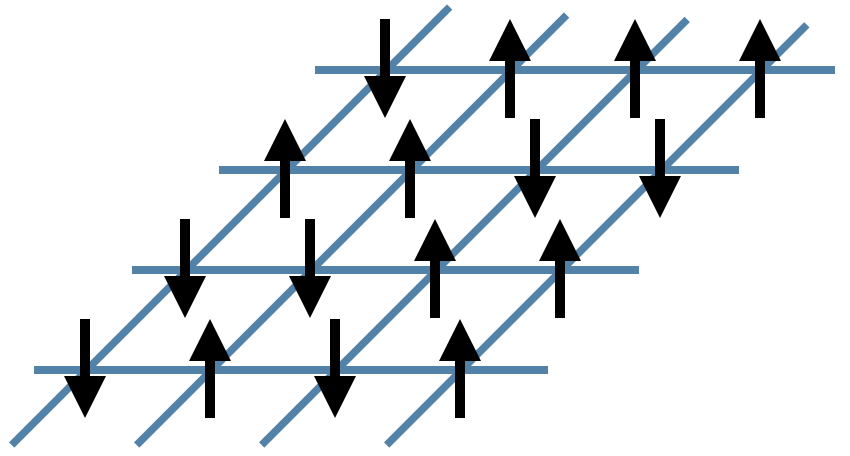In the figure above, the black arrows are the Ising spins $\sigma$ and the blue lines represent the local energies $\sigma_i \sigma_j$. The total energy $E$ of each configuration is the sum of all of these local energies.

Interestingly, it is again possible to rewrite the partition function sum $Z$ as a network of contracted tensors. Define the tensor $A^{\sigma_t \sigma_r \sigma_b \sigma_l}$ to be @@ A^{\sigma_t \sigma_r \sigma_b \sigma_l} = e^{-(\sigma_t \sigma_r + \sigma_r \sigma_b + \sigma_b \sigma_l + \sigma_l \sigma_t)/T} @@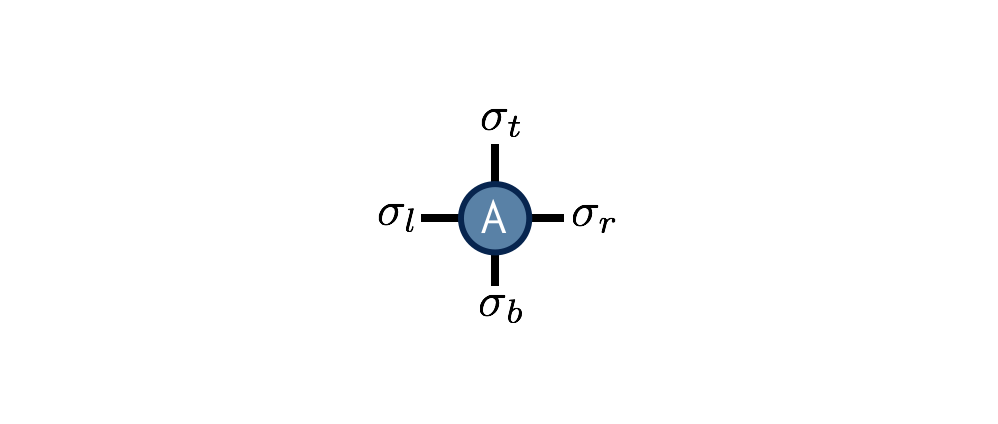The interpretation of this tensor is that it computes the local energies between the four spins that live on its indices, and its value is the Boltzmann probability weight $e^{-E/T}$ associated with these energies. Note its similarity to the one-dimensional transfer matrix $M$.

With $A$ thus defined, the partition function $Z$ for the two-dimensional Ising model can be found by contracting the following network of $A$ tensors: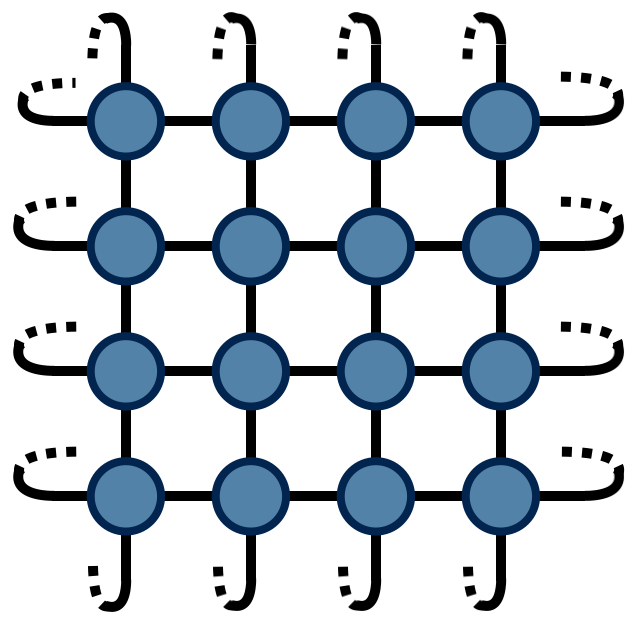The above drawing is of a lattice of 32 Ising spins (recall that the spins live on the tensor indices). The indices at the edges of this square wrap around in a periodic fashion because the energy function was defined using periodic boundary conditions.

## The TRG Algorithm

TRG is a strategy for computing the above 2d network, which is just equal to a single number $Z$ (since there are no uncontracted external indices). The TRG approach is to locally replace individual $A$ tensors with pairs of lower-rank tensors which guarantee the result of the contraction remains the same to a good approximation. These smaller tensors can then be recombined in a different way that results in a more sparse, yet equivalent network.

Referring to the original $A$ tensor as $A_0$, the first “move” of TRG is to factorize the $A_0$ tensor in two different ways: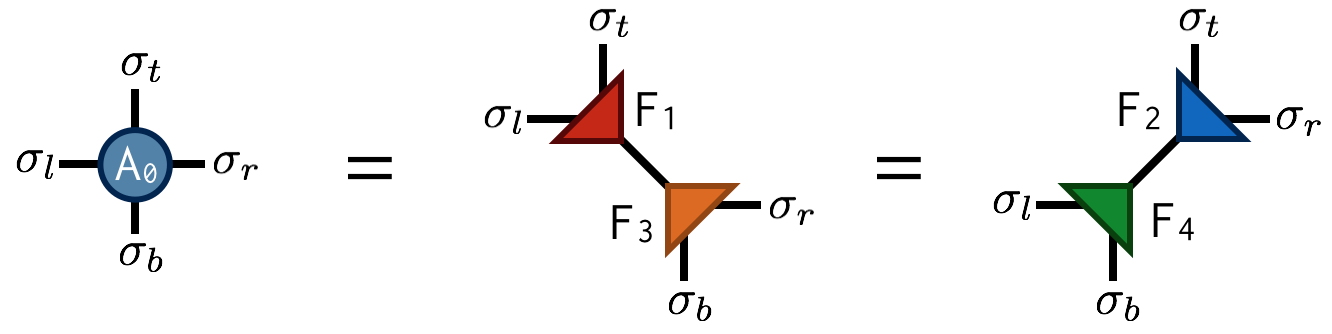Both factorizations can be computed using the singular value decomposition (SVD). For example, to compute the first factorization, view $A_0$ as a matrix with a collective “row” index $\sigma_l$ and $\sigma_t$ and collective “column” index $\sigma_b$ and $\sigma_r$. After performing an SVD of $A_0$ in this way, further factorize the singular value matrix $S$ as $S = \sqrt{S} \sqrt{S}$ and absorb each $\sqrt{S}$ factor into U and V to create the factors $F_1$ and $F_2$. Pictorially:Importantly, the SVD is only done approximately by retaining just the $\chi$ largest singular values and discarding the columns of U and V corresponding to the smaller singular values. This truncation is crucial for keeping the cost of the TRG algorithm under control.

Making the above substitutions, either $A_0=F_1 F_3$ or $A_0=F_2 F_4$ on alternating lattice sites, transforms the original tensor network into the following network: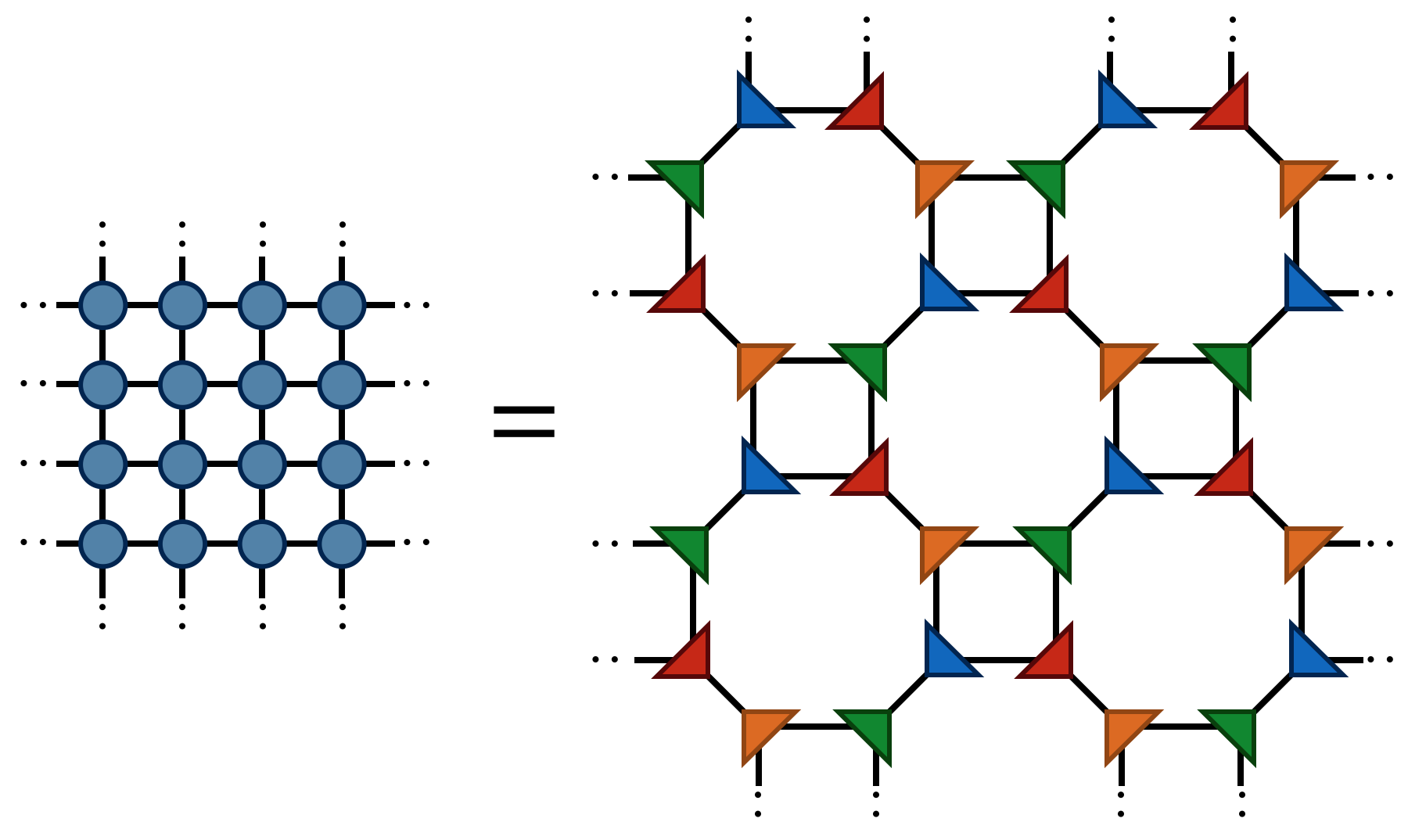Finally by contracting the four F tensors in the following way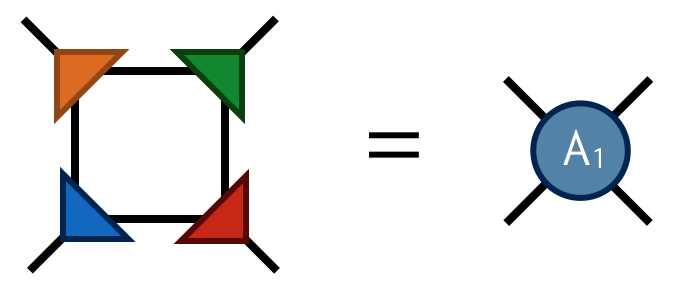one obtains the tensor $A_1$ which has four indices just like $A_0$. Contracting the $A_1$ tensors in a square-lattice pattern gives the same result (up to SVD truncation errors) as contracting the original $A_0$ tensors, only there are half as many $A_1$ tensors (each $A_0$ consists of two F’s while each $A_1$ consists of four F’s).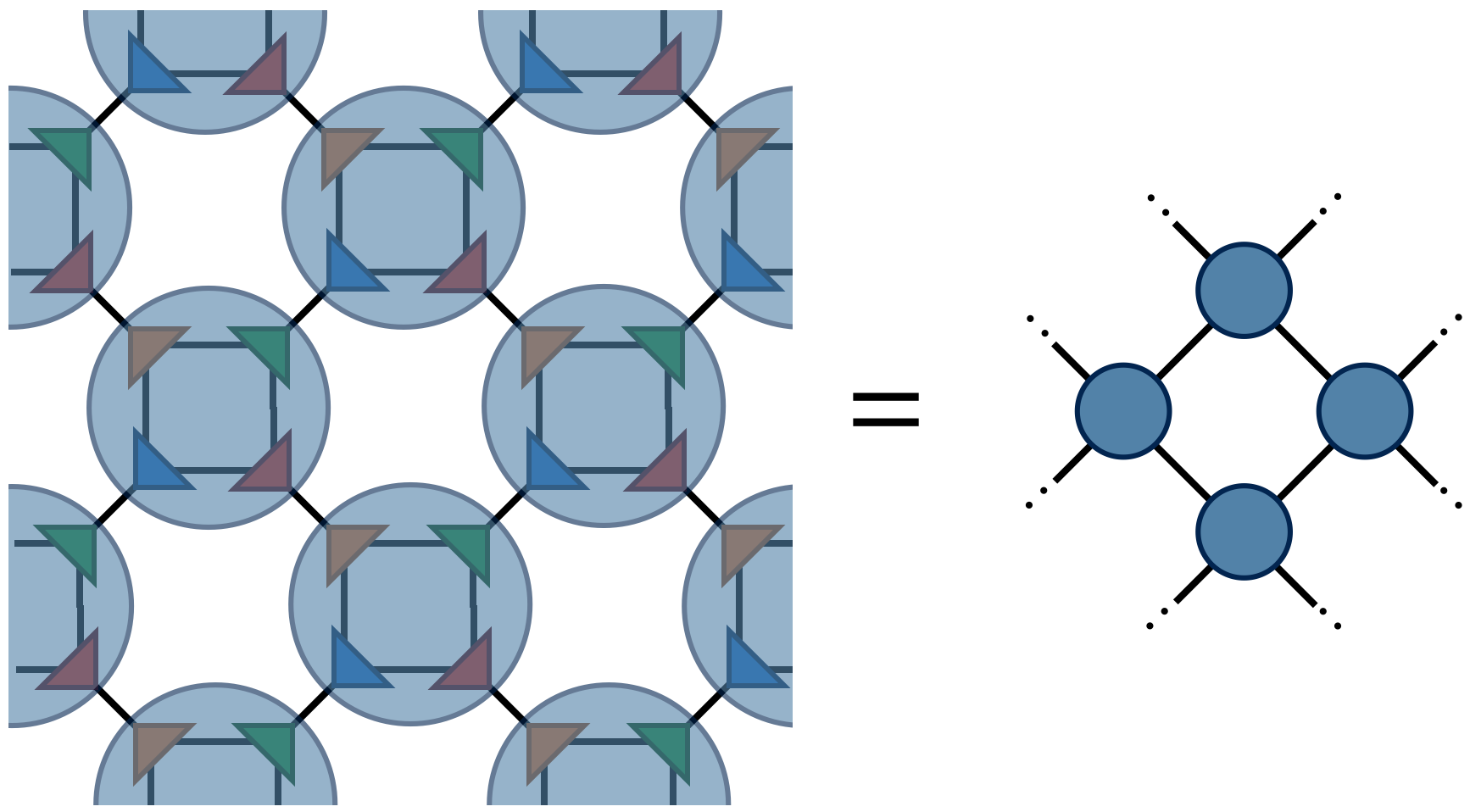To compute $Z$ defined by contracting a square lattice of $2^{1+N}$ tensors, one repeats the above two steps (factor and recombine) N times until only a single tensor remains. Calling this final tensor $A_N$, the result $Z$ of contracting the original network is equal to the following “double trace” of $A_N$:## Extensions

Extensions of the basic TRG algorithm include:

• tensor entanglement renormalization group (TERG) Ref. 2
• tensor network renormalization (TNR) Refs. 3, 4, 5
• loop-TNR Ref. 6
• GILT Ref. 7

• The original paper on TRG:

Levin and Nave, “Tensor Renormalization Group Approach to Two-Dimensional Classical Lattice Models”, PRL 99, 120601 (2007) cond‑mat/0611687

• Paper on TRG with very useful figures (particularly Fig. 5):

Gu, Levin, and Wen, “Tensor-entanglement renormalization group approach as a unified method for symmetry breaking and topological phase transitions” PRB 78, 205116 (2008) arxiv:0806.3509

• TNR is an extension of TRG which qualitatively improves TRG’s fixed-point behavior and can be used to generate MERA tensor networks:

Evenbly and Vidal, “Tensor Network Renormalization” PRL 115, 180405 (2015) arxiv:1412.0732

## References

1. Tensor Renormalization Group Approach to Two-Dimensional Classical Lattice Models, Michael Levin, Cody P. Nave, Phys. Rev. Lett. 99, 120601 (2007), cond‑mat/0611687
2. Tensor-entanglement renormalization group approach as a unified method for symmetry breaking and topological phase transitions, Zheng-Cheng Gu, Michael Levin, Xiao-Gang Wen, Phys. Rev. B 78, 205116 (2008), arxiv:0807.2010
3. Tensor Network Renormalization, G. Evenbly, G. Vidal, Phys. Rev. Lett. 115, 180405 (2015), arxiv:1412.0732
4. Algorithms for tensor network renormalization, G. Evenbly, Phys. Rev. B 95, 045117 (2017), arxiv:1509.07484
5. Tensor Network Renormalization Yields the Multiscale Entanglement Renormalization Ansatz, G. Evenbly, G. Vidal, Phys. Rev. Lett. 115, 200401 (2015), arxiv:1502.05385
6. Loop Optimization for Tensor Network Renormalization, Shuo Yang, Zheng-Cheng Gu, Xiao-Gang Wen, Phys. Rev. Lett. 118, 110504 (2017), arxiv:1512.04938
7. Renormalization of tensor networks using graph-independent local truncations, Markus Hauru, Clement Delcamp, Sebastian Mizera, Phys. Rev. B 97, 045111 (2018), arxiv:1709.07460